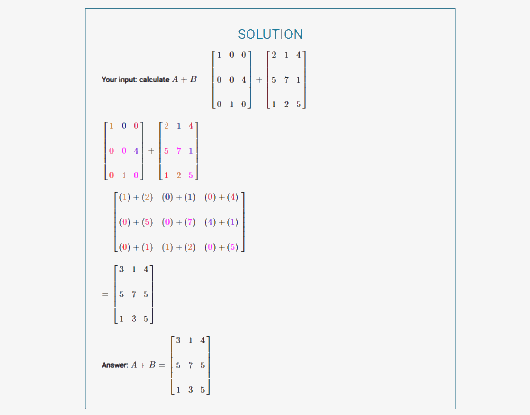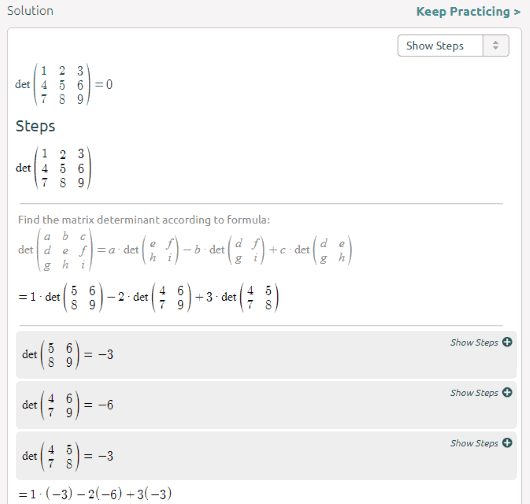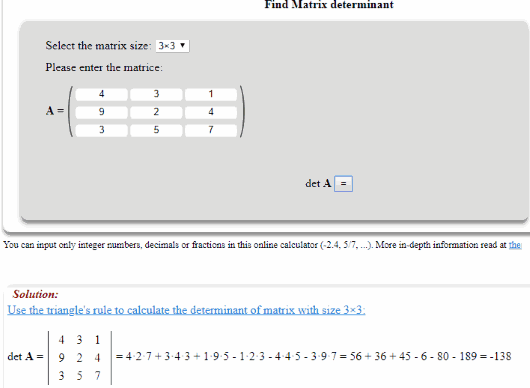Editor Ratings:
User Ratings:
[Total: 2 Average: 4]

Here are some good online matrix calculator with steps free websites. You can find inverse, determinant, addition, subtraction of matrices, matrix power, matrix multiplication, transpose, and more. All you need to do is add some values for the input matrix (or matrices) and then select the output operation, which could be the addition, transpose, subtraction, etc. After that, you will get the output answer in a box.

The steps related to how the input matrix was solved are also visible to you. While one website lets you expand individual steps separately, another website lets you see all the steps without expanding the steps manually.

Let’s check these free online matrix calculator with steps one by one.

## eMathHelpeMathHelp (Homepage) comes with a very useful Matrix Calculator tool. It lets you select an operation (addition, multiply, division, find inverse, determinant, raise to power, find rank, etc.), and add values to matrices. Once you have done that, you can use the Calculate button. The result is visible in a box. You can see the input matrices, steps how the matrix is solved, and the output matrix or answer.

If you want, you can also hide the output steps. From using this matrix solver to check the steps of output matrix, everything is very easy.

## SymbolabSymbolab website provides a separate Matrix Calculator feature where you can create a matrix from scratch or use the available examples. There are examples available for determinant, matrix subtraction, transpose, etc. Select an example, edit it, and then you can solve it and explore all the steps. To show all the steps, it prompts you to upgrade. However, you can explore individual steps one by one so that you won’t have to upgrade and see all the steps that needed to solve the matrix.

You can also save matrix equation ad steps as a PDF file to your PC. The output PDF will contain a watermark of website but it won’t be a problem for personal use.

## OnlineMSchoolOnlineMSchool brings matrix addition and subtraction, transpose, scalar multiplication, power, rank, determinant, inverse, and transpose matrix calculators. You can select any of the available tools and then add values to the matrix. After that, you need to click on the “=” button to get the output.

The output is visible as the answer as well as the steps of how that output was generated. Both, the output matrix and components (output steps), are visible in separate boxes. So, the clutter-free output is easy to understand.

### The Conclusion:

These are the free and simple online matrix calculator with steps. On this list, I find the first website better than others. Its simple interface, feature to select matrix dimensions and show/hide output steps make it a good website.

 Editor Ratings: User Ratings:[Total: 2 Average: 4] Tags: Matrix calculator